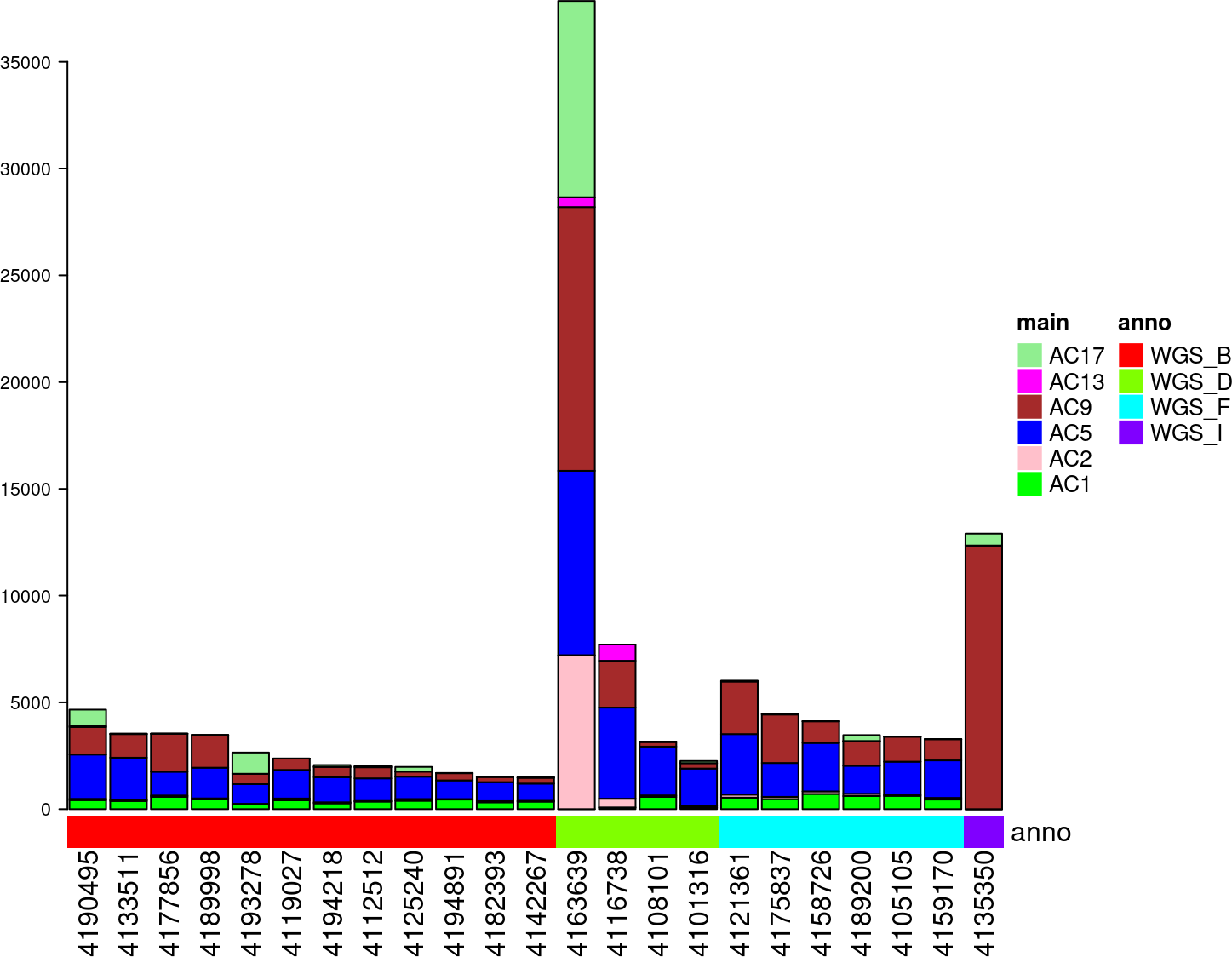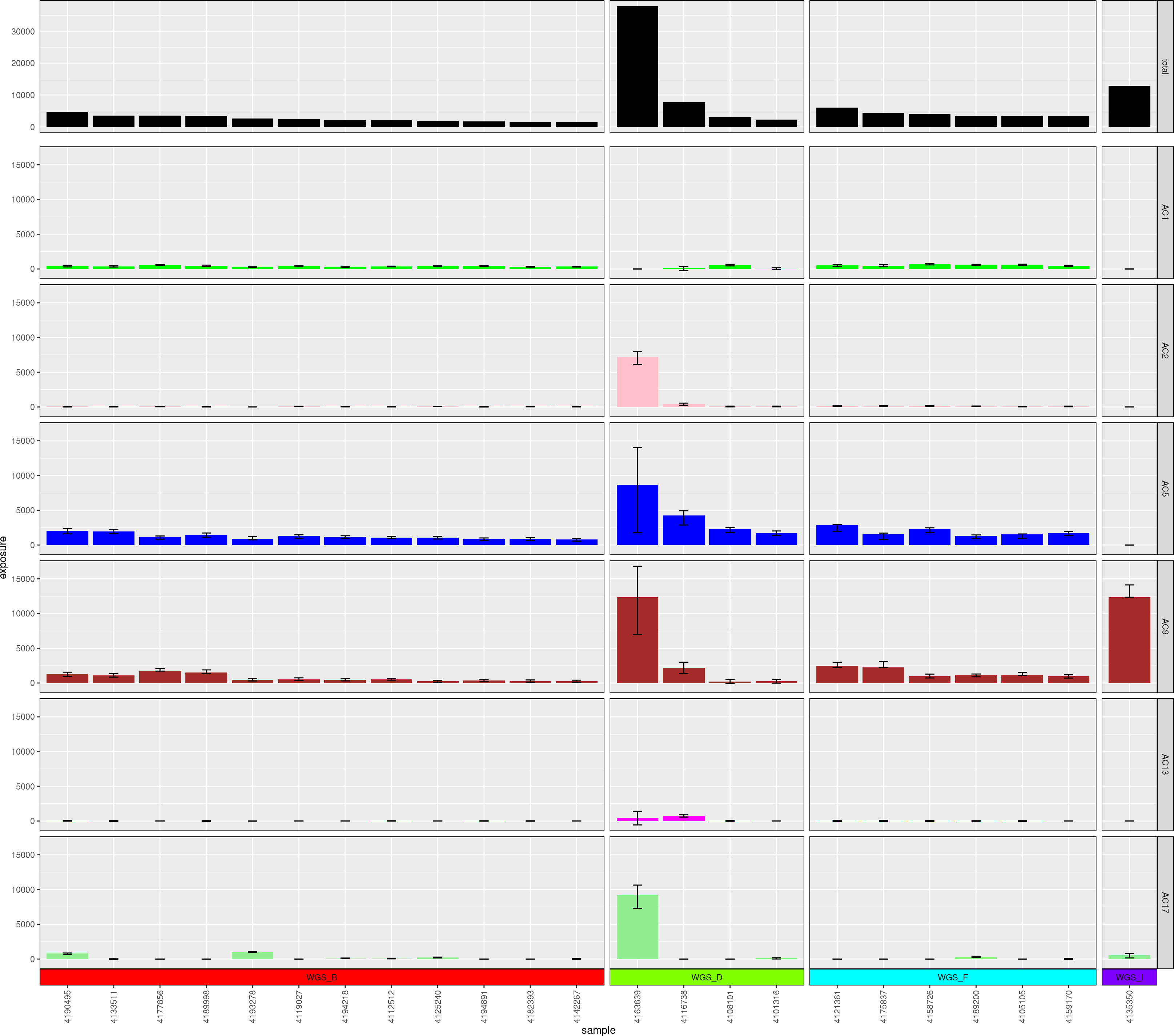# 1 Introduction: Confidence Intervals

In order to assess confidence in the setting of modeling in ordinary differential equations (ODEs), the concept of profile likelihood was introduced (Raue et al. 2009). In YAPSA, this concept was adapted to the computation of confidence intervals (CIs) for the exposures to mutational signatures (Alexandrov et al. (2013)). To determine the CI for a computed single value in a high-dimensional vector, this value is perturbed and the remaining values of the vector are computed again, yielding an alternative data model with one degree of freedom less than the initial model. Then, log-likelihoods are computed from the distribution of the residuals of the initial and the alternative model and a likelihood ratio test is being computed.

In the context of mutational signatures, this corresponds to the determination of the CI for the exposure of one given mutational signature exposure. To this end, this exposure value is perturbed, i.e., $$H_{uv}$$, the exposure to signature $$u$$ in sample $$v$$, is changed by a small value $$H_{uv} \rightarrow H_{uv} + \epsilon_{uv}$$, and the exposures to the remaining signatures are computed again by non-negative least squares, yielding an alternative data model with one degree of freedom less than the initial model. Then, as described above, log-likelihoods are computed from the distribution of the residuals of the initial and the alternative model and a likelihood ratio test is being computed. This yields a p-value for the perturbation, which may need to be extrapolated by a Gauss-Newton method to yield 95% CIs.

# 2 Recap: compute signature exposures

In the following section, we briefly recapitulate the analysis of SNV mutational signatures on an example data set as performed in 1. Usage of YAPSA. We thus first load the example data stored in the package:

data(sigs)
data(cutoffs)
data("lymphomaNature2013_mutCat_df")
current_cutoff_vector <- cutoffCosmicValid_abs_df[6,]

We then perform a supervised analysis of SNV mutational signatures using signature-specific cutoffs:

lymphoma_COSMIC_listsList <-
LCD_complex_cutoff_combined(
in_mutation_catalogue_df = lymphomaNature2013_mutCat_df,
in_cutoff_vector = current_cutoff_vector,
in_signatures_df = AlexCosmicValid_sig_df,
in_sig_ind_df = AlexCosmicValid_sigInd_df)

We assign subgroups to the different samples:

data(lymphoma_PID)
colnames(lymphoma_PID_df) <- "SUBGROUP"
lymphoma_PID_df$PID <- rownames(lymphoma_PID_df) COSMIC_subgroups_df <- make_subgroups_df(lymphoma_PID_df, lymphoma_COSMIC_listsList$cohort$exposures) And finally plot the obtained result: exposures_barplot( in_exposures_df = lymphoma_COSMIC_listsList$cohort$exposures, in_signatures_ind_df = lymphoma_COSMIC_listsList$cohort$out_sig_ind_df, in_subgroups_df = COSMIC_subgroups_df)Figure 1: Exposures to SNV mutational signatures # 3 Compute the confidence intervals In order to assess trustworthiness of the computed exposures, YAPSA provides the calculation of CIs. Analogously to CIs for SNV mutational signatures, the CIs for Indel mutational signatures are computed using the concept of profile likelihood. This is performed by the function variateExp(). complete_df <- variateExp( in_catalogue_df = lymphomaNature2013_mutCat_df, in_sig_df = lymphoma_COSMIC_listsList$cohort$signatures, in_exposures_df = lymphoma_COSMIC_listsList$cohort$exposures, in_sigLevel = 0.025, in_delta = 0.4) Of note and as opposed to the output of the LCD function family, the result of the function variateExp() is a data frame in a long format, because for every combination of a signature and a sample, several values now have to be stored: head(complete_df, 12) ## sig sample exposure relLower relUpper lower upper ## 1 AC1 4101316 72.675768 -0.43106098 2.374512 -31.327688 172.56949 ## 2 AC2 4101316 74.489434 0.34878710 1.680106 25.980954 125.15014 ## 3 AC5 4101316 1753.476803 0.77488620 1.152261 1358.744975 2020.46228 ## 4 AC9 4101316 255.812357 -0.07894218 1.995232 -20.194385 510.40496 ## 5 AC13 4101316 0.000000 0.00000000 0.000000 0.000000 0.00000 ## 6 AC17 4101316 97.545639 0.05837721 1.898057 5.694443 185.14718 ## 7 AC1 4105105 620.579640 0.80801718 1.100735 501.439012 683.09381 ## 8 AC2 4105105 62.117385 0.34583451 1.768123 21.482335 109.83116 ## 9 AC5 4105105 1538.699883 0.62467887 1.032297 961.193310 1588.39561 ## 10 AC9 4105105 1171.315692 0.91125235 1.316790 1067.364176 1542.37690 ## 11 AC13 4105105 6.287399 -7.75516430 7.314664 -48.759815 45.99022 ## 12 AC17 4105105 0.000000 0.00000000 0.000000 0.000000 0.00000 Here, the column exposure contains the values which had been computed before. The columms relLower and relUpper contain the factors with which to multiply the exposures in order to get the lower and upper bounds of the 95% CIs. The absolute values of these lower and upper bounds are stored in the columns lower and upper. There also is a custom function to plot exposures with confidence intervals: plotExposuresConfidence( in_complete_df = complete_df, in_subgroups_df = COSMIC_subgroups_df, in_sigInd_df = lymphoma_COSMIC_listsList$cohort\$out_sig_ind_df)Figure 2: Confidence interval calculation for exposures to mutational
signatures

This produces a figure similar to the display of exposures obtained above, but in contrast to this former way of displaying signature exposures by stacked barplots, here we chose a facet plot with the signatures as rows in order to be able to display the CIs, which are indicated as whiskers. We furthermore would like to emphasize that if a signature is not present in a sample, i.e., the exposure to that signature is 0, then the upper and lower bounds of the confidence interval are zero as well.

Of note, the functionality to compute 95% CIs for signature exposures is also available for the analysis of Indel mutational signatures, an example is provided in the corresponding vignette.